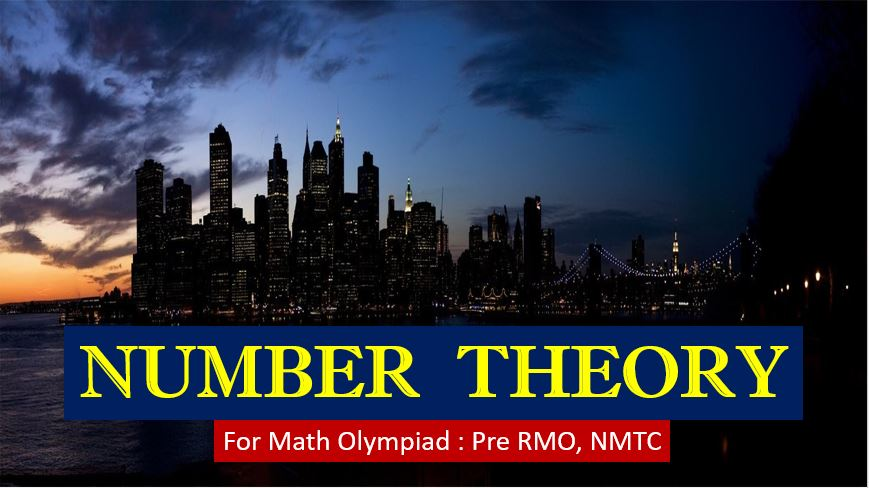Saturday, May 30, 2020
Home > IMO > Number Theory Math Olympiad Syllabus

# Number Theory Math Olympiad SyllabusHi students, welcome to Amans Maths Blogs (AMB). You are on this post Number Theory Olympiad Math Olympiad Syllabus, it means you preparing for some important exams like Pre-RMO, RMO, INMO, IMO and NMTC which are based on MATH subject only.

These exams require depth knowledge of Math subject in specially in its syllabus like Number System, Theory of Equations, Combinatorics, Probability, Functions, Algebra, Geometry etc.

We learn these concepts as one by one with depth knowledge of its subtopics.

# Number Theory

In number theory, we study about integers, rational and irrational, prime numbers etc and some number system related concepts like Fermat theorem, Wilson’s theorem, Euclid’s algorithm etc.

According to Wikipedia, “Number Theory is a branch of Pure Mathematics devoted primarily to the study of integers. Number Theory is the queen of mathematics“.

Now, we need to learn the following concepts of number theory which are in the syllabus of Math Olympiad level exams.

## Number System

In number system, we need to study about the numbers, types of numbers like natural numbers, whole numbers, integers, fractions, rational and irrational numbers etc. We also learn about the properties of numbers.

## Divisibility Rules

In divisibility rules, we will learn about the test of divisibility of integers. It means, what are the conditions of divisibility by 2, 3, 4, 5, 6, 7, 8, 9, 10, 11 13 17 etc. We will also learn the proofs of these divisibility conditions and we also cover the properties of divisibility.

## Division Algorithm

In division algorithm, we will study about the division of two natural numbers and more details about its remainder. In this, we know about the relationship between the dividend, divisors and the remainders.

## Greatest Common Factor (GCF or HCF)

In greatest common factor, we will get the knowledge about the GCF or HCF of the two positive integers and their properties. We will also learn how to find the HCF of two numbers.

## Latest Common Multiple (LCM)

In least common multiple, we will study about the LCM of the two positive integers and their properties. We will also learn how to find the LCM of two numbers.

## Prime Numbers

In this, we study about some important numbers like prime numbers and we will also learn about their properties of prime numbers related to divisibility.

## Coprime Numbers

In this, we study about some important numbers like coprime numbers and we will also learn about their properties of coprime numbers.

## Euler’s Function

In this, we will learn to find the number coprime numbers less than a number.

## Composite Numbers

In this, we study about some important numbers like composite numbers and we will also learn about their properties of composite numbers.

## Sophie German Identity (SGI)

In Sophie German Identity, we will learn to check whether a given number is a prime or composite.

## Euclidean Algorithm

In Euclidean algorithm, we will study the procedure of finding the HCF of two integers and we also learn how to represent the HCF of two numbers into the given numbers

## Prime Factorization

The prime factorization of a number is also known as the fundamental theorem of arithmetic. In this, we will learn about to find the prime factorization of a number and some basic result based on it like number of divisors, number of even divisors and sum and product of divisors etc.

## Congruence Modulo

In congruence modulo, we study about a special property of a number which help us to find last digit, last two digit etc and some remainder in the division of two numbers

## Greatest Integer Function (GIF)

In this, we study greatest integer function of a number which is used to find the nearest integer less than the number and we also learn about the properties of greatest integer function of a number.

## Fermat Theorem

In this, we study about Fermat theorem and Fermat Little Theorem and its proof and some basic questions asked based on this theorem.

## Wilson Theorem

In this, we study about Wilson’s theorem and its proof and some basic questions asked based on this theorem.

## Chinese Remainder Theorem (CRT)

In this, we study about Chinese remainder theorem and its proof and some basic questions asked based on this theorem.

## Digits Concepts

In digit concepts, we will learn to find the sum of digits in expansion of xn and how to find the last digit, las two digits, last three digit etc.

## Factorial

In factorial, we will learn how to find the factorial of a number and we will also study about the some properties of factorial and some basic questions based on it.

## Exponents in n!

In this, we will learn how to find the exponents of a prime in prime factorization of factorial of a number.

## Successive Division

In successive division, we will study about the division of numbers and need to find the remainders etc